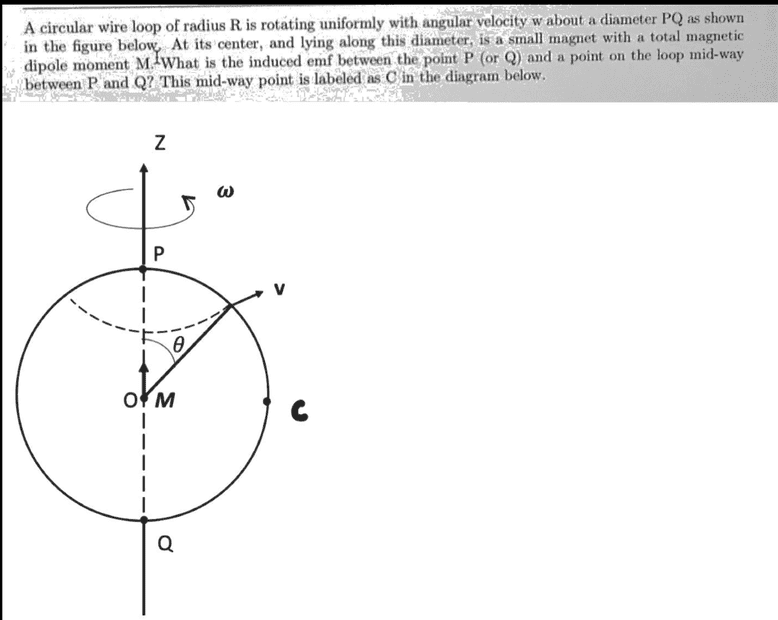# EMF induced in a wire loop rotating in a magnetic field

• LCSphysicist
In summary, the conversation discusses the problem of evaluating the integral for the electric field in a configuration involving a rotating sphere and a time-independent magnetic dipole. The discussion includes the concept of motional emf and the relationship between the Lorenz force, charge separation, and electric field. The equilibrium condition is also mentioned as a factor in determining the electric field in this situation.

#### LCSphysicist

Homework Statement
.
Relevant Equations
.To solve this problem, we need to evaluate the following integral: $$\epsilon = \int_{P}^{C} (\vec v \times \vec B) \vec dl$$

The main problem is, in fact, how do we arrive at it! I can't see why a Electric field arises at the configuration here. The magnetic field of the rotating sphere is time independent ##(\frac{ d \omega }{dt}) = 0##. The magnetic dipole at the center is also time independent.

So why do a electric field arise? Worst: Why do it arise and is equal to ##\vec v \times \vec B##?

Have you studied motional emf?

kuruman said:
Have you studied motional emf?
It is more about the difficult to see that the area to calculate the flux is constant in avarage, but in infinitesimally time it is varying?

Herculi said:
So why do a electric field arise? Worst: Why do it arise and is equal to ##\vec v \times \vec B##?

The Lorenz force ##F_L=(v\times B)q## makes the electrons move, and this creates charge separation or simply charge density ##\rho\neq 0##. This charge density creates electric field according to Gauss's law $$\nabla\cdot \mathbf{E}=\rho$$.
How do we know that the line integral of this electric field equals to $$\int_C^P \mathbf{E}\cdot d\mathbf{l}=\int_C^P(\mathbf{v}\times\mathbf{B})\cdot d\mathbf{l}$$.

Well we also impose the additional equilibrium condition $$F_E=F_B\Rightarrow \mathbf{E}q=-(\mathbf{v}\times\mathbf{B})q$$

I am feeling we 'll have to open some can of worms if we going to discuss why this equilibrium condition holds but anyway that is my take on this problem.

P.S In my opinion the equilibrium condition holds approximately in the quasi static approximation, that is when B and v are independent of time or vary slowly in time so that the charges move to the equilibrium position almost instantaneously.

Last edited: